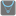• # Cannot pass : abs(i) for i in range(-10, 10)

## Question related to missionMin and Max

Hello,

I recently started checkio and am trying the task "Min and Max". For the test of "min(abs(i) for i in range(-10, 10))", I got following error message.

TypeError: <class 'dict'> is wrong data type

In this case, I understand the args was passed as generator object. I expected that each element (10, 9, 8, 7, ....) in the generator object was picked up in the block for statement (for elem in args: in my code), however, it seemed that the local variable (elem) stored generator object itself. One strange thing is that the code works fine when I simulated in my local python 3.5.2 environment.

Can anyone give me some hints to moving forward?

Best regards,

My source code is here:

```def min(*args, **kwargs):

key = kwargs.get("key", None)

if type(args) is list:
args = tuple(args)
elif type(args) is str or type(args) is range:
args = tuple(list(args))
elif type(args) is tuple:
args = args

temp_min = None

for elem in args:
if key is not None:
if temp_min == None:
temp_min = elem
elif key(temp_min) > key(elem):
temp_min = elem
else:
if temp_min == None:
temp_min = elem
elif temp_min > elem:
temp_min = elem

return temp_min

def max(*args, **kwargs):
key = kwargs.get("key", None)

if type(args) is list:
args = tuple(args)
elif type(args) is str or type(args) is range:
args = tuple(list(args))
elif type(args) is tuple:
args = args

temp_max = None

for elem in args:
if key is not None:
if temp_max == None:
temp_max = elem
elif key(temp_max) < key(elem):
temp_max = elem
else:
if temp_max == None:
temp_max = elem
elif temp_max < elem:
temp_max = elem

return temp_max

if __name__ == '__main__':
#These "asserts" using only for self-checking and not necessary for auto-testing
assert max(3, 2) == 3, "Simple case max"
assert min(3, 2) == 2, "Simple case min"
assert max([1, 2, 0, 3, 4]) == 4, "From a list"
assert min("hello") == "e", "From string"
assert max(2.2, 5.6, 5.9, key=int) == 5.6, "Two maximal items"
assert min([[1, 2], [3, 4], [9, 0]], key=lambda x: x) == [9, 0], "lambda key"
print("Coding complete? Click 'Check' to review your tests and earn cool rewards!")
```9